# waynehu

## Professor, Department of Astronomy and Astrophysics University of Chicago

Group Contact CV SnapShots

## Data Analysis

Several authors have addressed the question of the optimal estimators of the polarization power spectra from high sensitivity, all-sky maps of the polarization. They suggest that one calculate the coefficients of the expansion in spin-2 spherical harmonics and then form quadratic estimators of the power spectrum, as the average of the squares of the coefficients over m, corrected for noise bias as in the example of Fig. 14.

We shall return to consider this below, however before such maps are obtained we would like to know how to analyze ground based polarization data. These data are likely to consist of Q and U measurements from tens or perhaps hundreds of pointings, convolved with an approximately gaussian beam on some angular scale. How can we use this data to provide constraints or measurements of the electric and magnetic power spectra, presumably averaged across bands in?

For a small number of points, the simplest and most powerful way to obtain the power spectrum is to perform a likelihood analysis of the data. The likelihood function encodes all of the information in the measurement and can be modified to correctly account for non-uniform noise, sky coverage, foreground subtraction and correlations between measurements. Operationally, one computes the probability of obtaining the measured points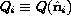and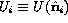assuming a given ``theory'' (including a model for foregrounds and detector noise) and maximizes the likelihood over the theories. For our purposes, the theories could be given simply by the polarization bandpowers in E and B for example, or could be a more ``realistic'' model such as CDM with a given reionization history. The confidence levels on the parameters are obtained as moments of the likelihood function in the usual way. Such an approach also allows one to generalize the analysis to include temperature information (for the cross correlation) if it becomes available.

Assuming that the fluctuations are gaussian, the likelihood function is given in terms of the data and the correlation function of Q and U for any pair of the n data points. The calculation of this correlation function is straightforward, and [Kamionkowski et al.] (1997) discuss the problem extensively. Let us assume that we are fitting only one component or have only one frequency channel. The generalization to multiple frequencies with a model for the foreground is also straightforward. We shall also assume for notational simplicity that we are fitting only to polarization data, though again the generalization to include temperature data is straightforward. The construction is as follows. We define a data vector which contains the Q and U information referenced to a particular coordinate system (in principle this coordinate system could change between different subsets of the data). Call this data vectorwhich has N=2n components. We can construct the likelihood of obtaining the data given a theory once we know the correlation matrix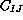: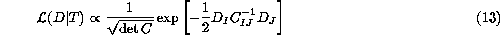All of the theory information is encoded in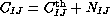, where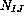is the noise correlation matrix, to be provided by the experiment, and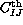is a function of the theory parameters.

All that remains is to compute each element offor a given theory. Consider a pair of points i and j corresponding to 4 entries of our data vector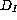. Following [Kamionkowski et al.] (1997), define Q' and U' as the components of the polarization in a new coordinate system, where the great arc connecting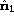and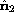runs along the equator. Expressions for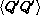and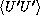in terms of the E and B angular power follow directly from the definitions of these spectra and the spin-weighted spherical harmonics. They can be found in [Kamionkowski et al.] (1997) [their Eqs. (5.9,5.10)]. They also give the flat sky limit of these equations. Knowing the angle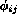about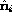through which we must rotate our primed coordinate system to return to the system in which our data is defined we can write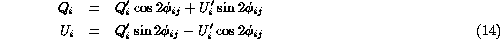and similarly for the jth element. Thus using the known expressions forandwe can calculate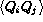,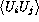,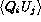and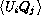for the ith and jth pixel and thus all of the elements of. Substitution ofinto Eq. (13) allows one to obtain limits on any theory given the data.

Finally let us note that the method outlined here is completely general and thus can be applied also the the high-sensitivity, all-sky maps which would result from satellite experiments. For these experiments, the large volume of data is however an issue in the analysis pipeline design, as has been addressed by several authors. The generalization of the above analysis procedure to include filtering and compression is straightforward, and directly analogous to the case of anisotropy, so we will not discuss it explicitly here.

Next: Future Prospects